# How to Only Sum Visible Cells/Rows in a Filtered List in Excel

Sometimes we may want to only sum the visible cells in a filtered list or a table. We may notice that if we use SUM function directly with selecting the visible reference in the filtered list, all data in the list (also contains the hidden rows) is included into calculation.

Above all, we need to find a proper function can only sum visible cells in a filtered list and ignore the filtered-out data. Actually, excel built-in SUBTOTAL function can perform calculating like sum data, count, average for both visible & invisible cells or only visible cells properly. As we only want to show you the formula to perform sum visible cells in filter list, so in this article we will only introduce you the basic usage of SUBTOTAL function on sum data. After reading this article, I think you must have a well understanding of SUBTOTAL function, it is flexible for you to use it in your daily work.

EXAMPLE: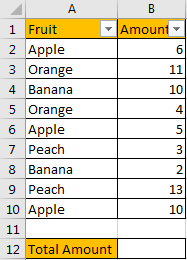If we want to sum total amount for the filtered fruit, can we use SUM function here directly?

Enter =SUM(B2:B10) in B12. See screenshot below, we get 64 here, the returned value is incorrect obviously. It equals to the total amount of the whole list B2:B10, the hidden rows are also taken into calculation.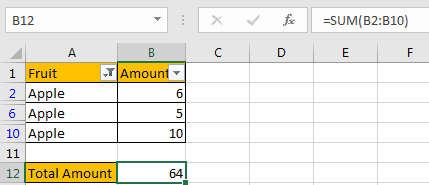FORMULA:

Let’s apply SUBTOTAL function in this case to try to calculate total amount for ‘Apple’ again.

Step 1: Enter =SUBTOTAL(9,B2:B10).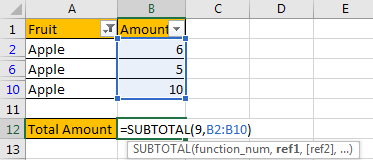Step 2: Press Enter to load return value.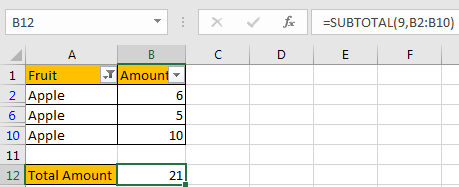We can see that this time correct amount 21 is displayed in B12.

HOW THIS FORMULA WORKS:

This formula only contains only one function: SUBTOTAL function.

Syntax:

=SUBTOTAL (function_num, ref1, [ref2], …)

It has several arguments like function_num, ref1, ref2. For function_num, it is a number, it shows the function used by SUBTOTAL, by providing different numbers, SUBTOTAL function can perform different calculation. As we mentioned above, SUBTOTAL function can perform most basic calculations like SUM, COUNT, AVERAGE, etc., so we need to know some useful function numbers before explaining SUBTOTAL function.

Please see below screenshots, they show the correspondence between number and function.

Function number from 1 – 101.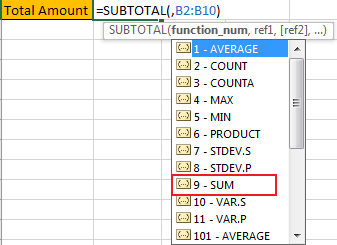Function number from 101 – 111.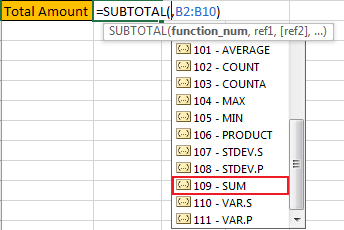Refer to above function number list, we can see that SUM function has two function numbers, 9-SUM and 109-SUM. Actually, if we enter 9, SUBTOTAL function will only sum visible data and ignore the filtered-out data; and if we enter 109, SUBTOTAL function will only sum visible data and ignore the manually hidden data. See, the difference is:

9: ignore data which is hidden by filter list;

109: ignore data which is hidden manually.

In this instance, in the formula =SUBTOTAL(9,B2:B10). There are two arguments. For the first argument function_num, as data is hidden by filter list, so we enter 9 here; for the second argument, reference, we can enter the whole list B2:B10, or you can also hold your mouse and select the visible cells directly.

RESULT:

We already get proper amount for ‘Apple’, this time let’s pick Banana to check if our formula works properly after changing the filter criteria.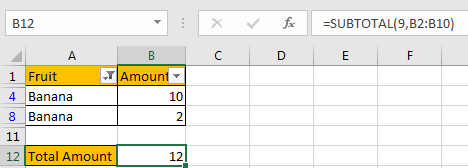It works properly. SUBTOTAL function can work well on ‘sum visible cells’.

NOTES:

1. If we change function_num from 9 to others, the SUBTOTAL function will change calculation accordingly based on the function number. For example, change 9 to 2 in this case (2 – COUNT).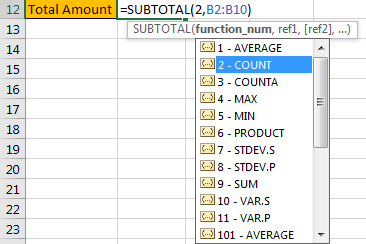We will get 2 by applying the formula.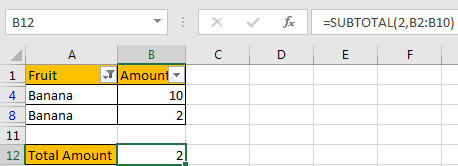1. SUBTOTAL function can ignore all hidden data, so we can also use it to sum visible cells in a range. Just enter the range as the reference, the it will ignore hidden cells in this range.

### Related Functions

• Excel SUBTOTAL function
The Excel SUBTOTAL function returns the subtotal of the numbers in a list or database. The syntax of the SUBTOTAL function is as below:= SUBTOTAL (function_num, ref1, [ref2])….
• Excel SUM function
The Excel SUM function will adds all numbers in a range of cells and returns the sum of these values. You can add individual values, cell references or ranges in excel.The syntax of the SUM function is as below:= SUM(number1,[number2],…)…

Related Posts

If Cell Contain Specific Text

This article will explain how to check if a cell contains text or a specific text string in Google Sheets or Microsoft Excel spreadsheets. In our daily work, we often look for specific text in worksheets containing large amounts of ...

Free Family Budget Template (Step-by-step Guide)

This article will show you some free printable family budget templates for google sheet and Microsoft Excel spreadsheet applications and will also explain some of the features or important functions of these templates. This will make it easy for you ...

6 Best Free Wedding Budget Templates

This post will show you some free wedding budget spreadsheet templates for both google sheets and Microsoft Excel Spreadsheet, as well as some explanations of the features or important function points of these templates. You can then easily make modifications ...

Calculate Cumulative Totals with Excel SUM Function

Today, through a simple example, we will show you how to use one of the most common-used Mathematical functions in excel, the SUM function, to add up the sum. In our daily life, we keep an account of what we ...

Check Dates in chronological order

Assume you have a date list that has different date formats, as seen in the accompanying picture. In this instance, Excel's Sort function will fail to sort them appropriately. However, you may convert all various date formats to a particular ...

Sidebar